Sign in# Chemistry Revision: Introduction to Organic Chemistry 1

What is organic chemistry?

Organic chemistry is the branch of chemistry that deals with organic molecules.

What is an organic molecule?

An organic molecule is one which contains carbon,

What are the main Elements in an organic compound?

main element in organic compounds is

carbon, and other elements include

Hydrogen (H), Oxygen (O), Nitrogen (N), Sulphur (S) and Phosphorus (P)

Unique property of carbon responsible for organic molecules formation.

Carbon has four valence electrons which means that each carbon atom can form bonds with four other atoms.

this what is responsible for, long chain structures and organic compounds.

A long chain structure of organic compounds can either be unbranched or branched

Branched Carbon chain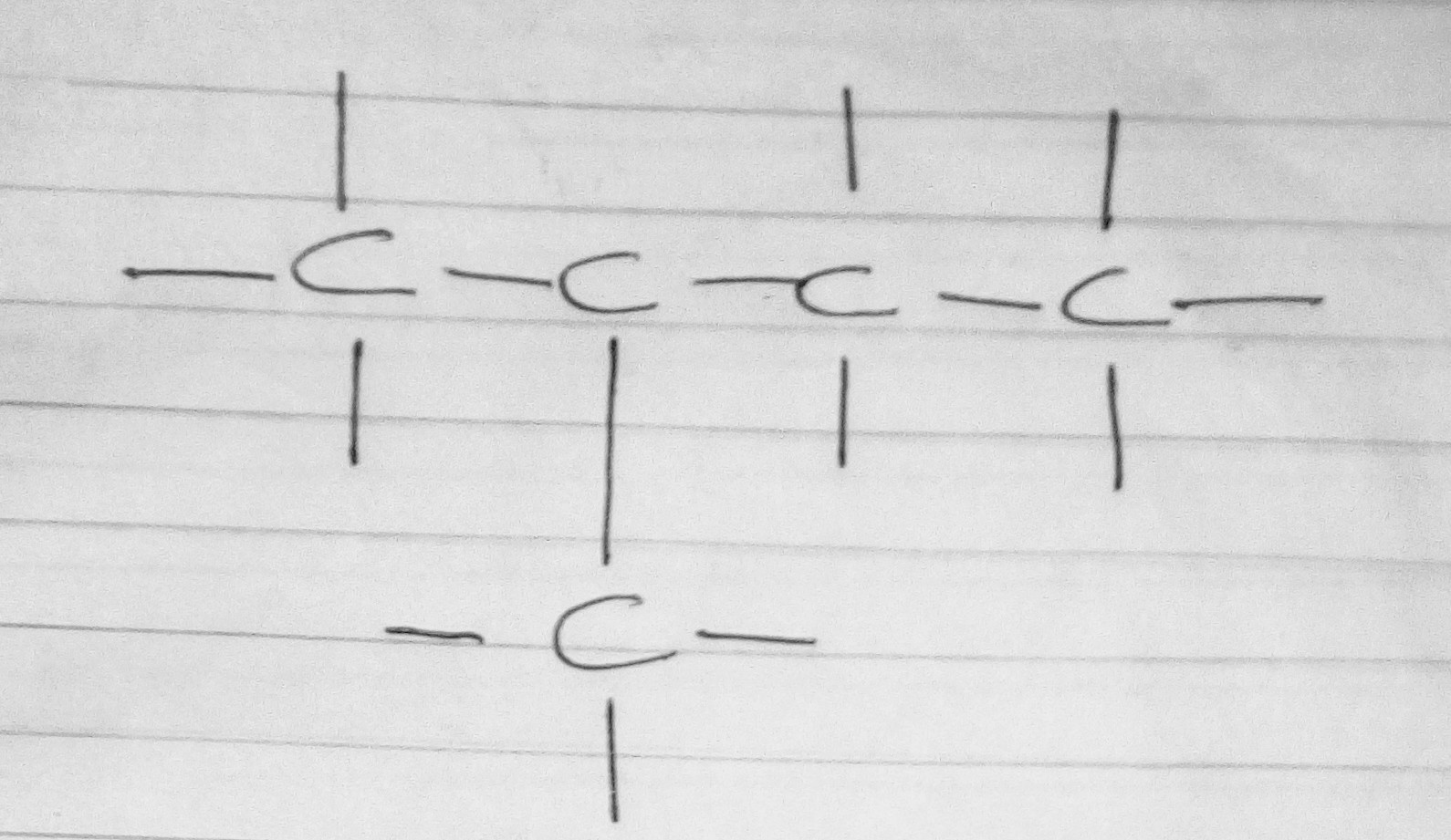unbranched Carbon chain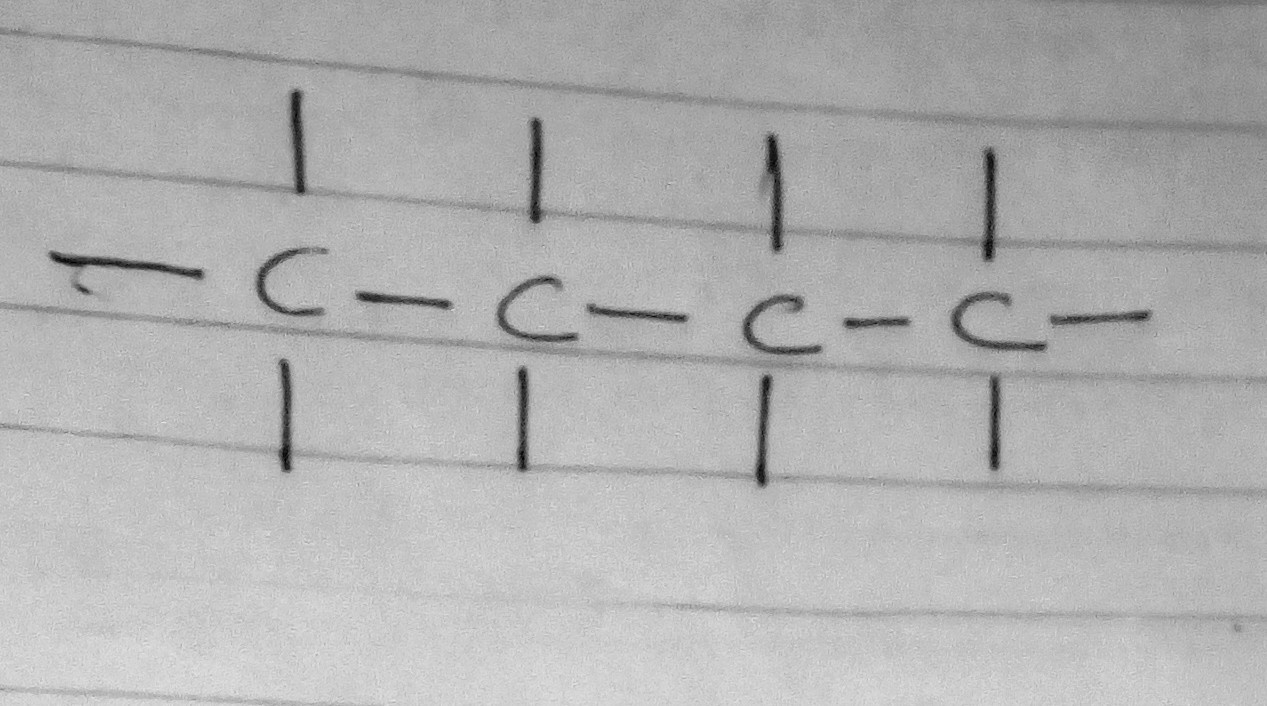How to Represent an Organic Compound

There are 3 main ways to represent an organic compound

Molecular formula, Structural formula, Condensed structural formula.

For clarity, I shall use 2-methylpropane as an example.

Molecular formula

The molecular formula of a compound shows how many atoms of each element are in a molecule of a compound.

For example, in 2-methylpropane, there 4 are atoms of carbon and 10 atoms of hydrogen thus, the molecular formula should look like what you are seeing, on the image below.Structural formula

The structural formula of an organic compound shows every bond between every atom in the molecule.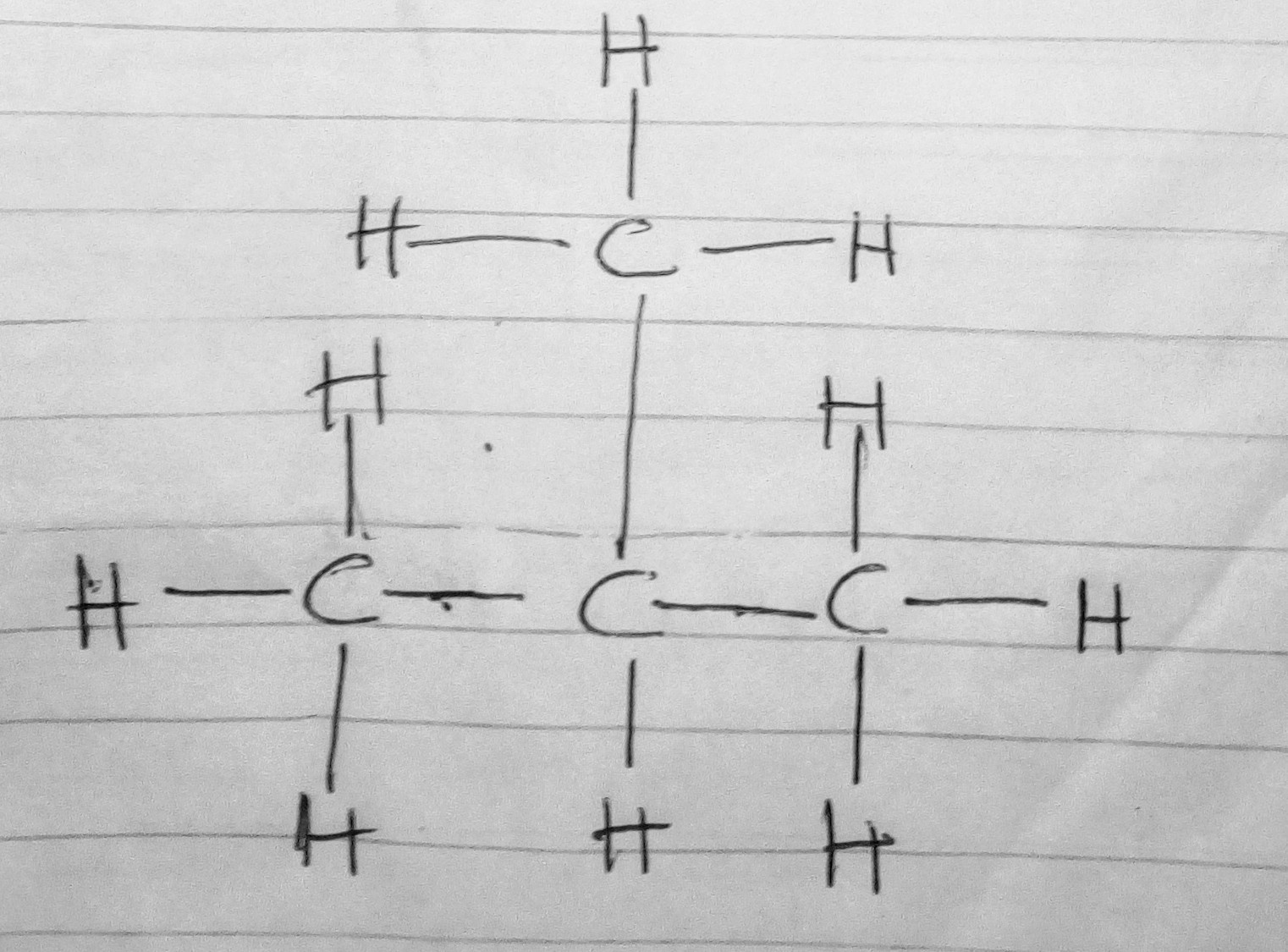Condensed structural formula.

When a compound is represented using its condensed structural formula, each carbon atom and the hydrogen atoms that are bonded directly to it are listed as a molecular formula, followed by a similar molecular formula for the neighboring carbon atom. Branched groups are shown in brackets after the carbon atom to which they are bonded to. The condensed structural formula below shows that in 2-methylpropane,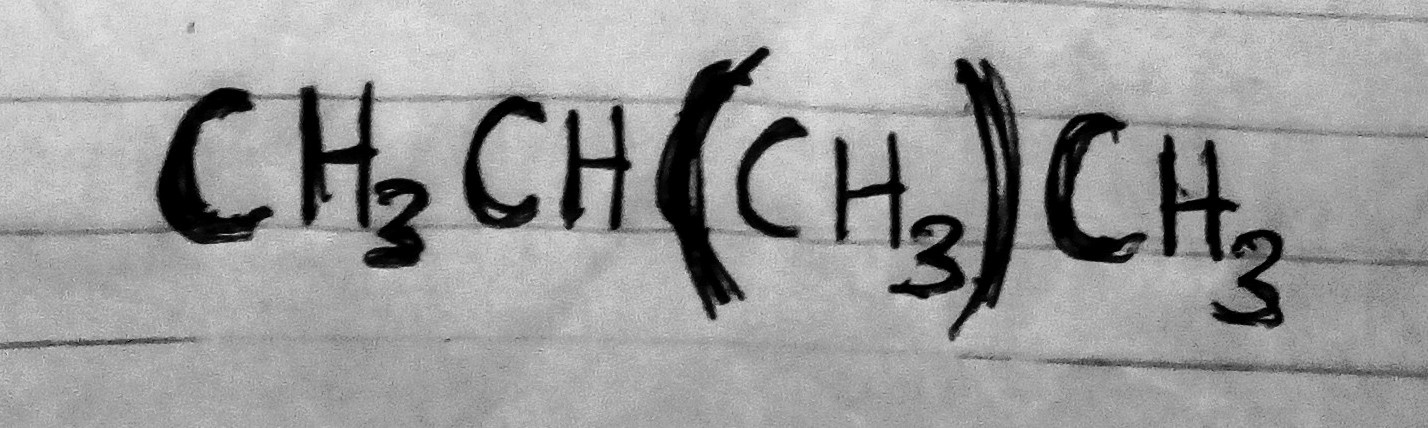Practice these questions and pass organic chemistry.

1) For each of the following, give the structural formula and the molecular formula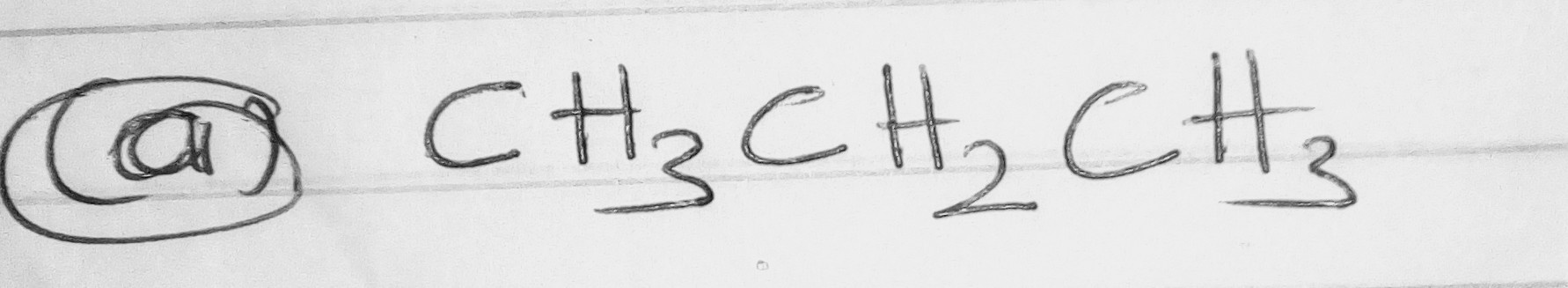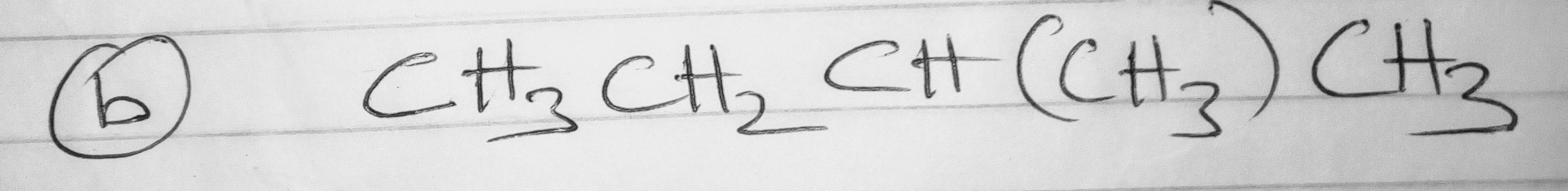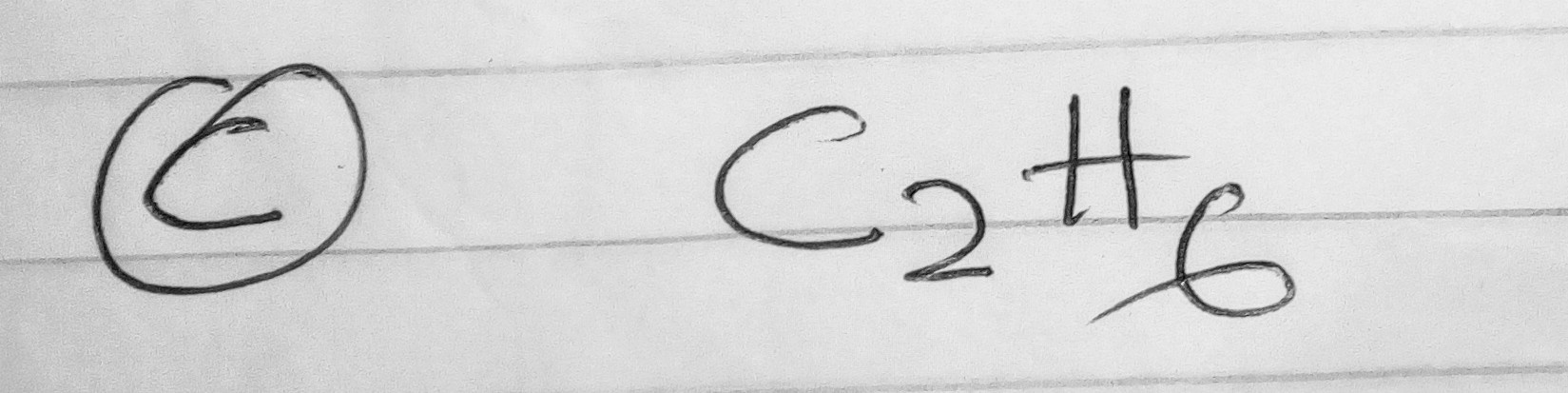2) Give two possible structural formulae for the compound with a molecular formula of3) For each of the following organic compounds, give the condensed structural formula and the molecular formula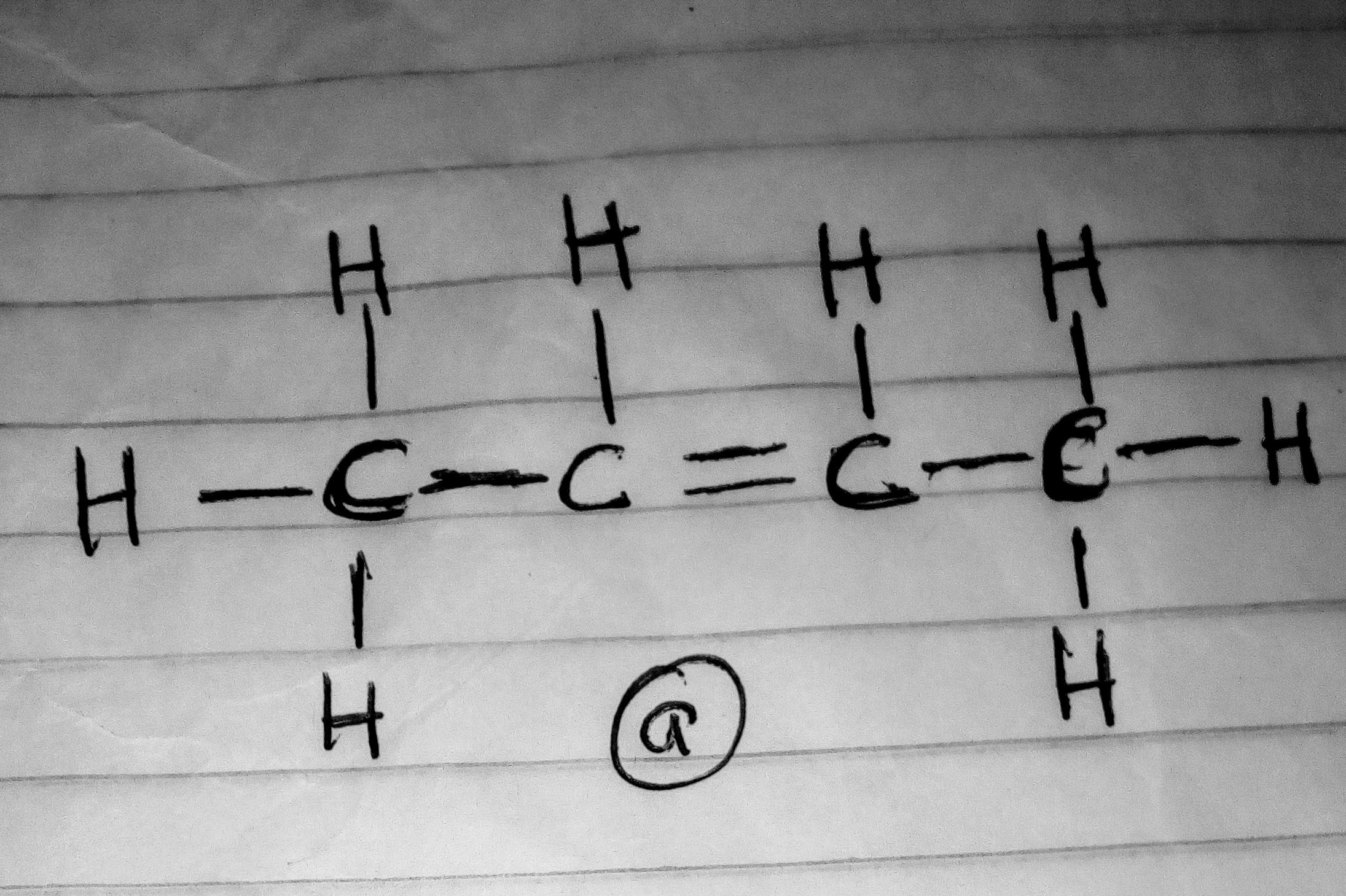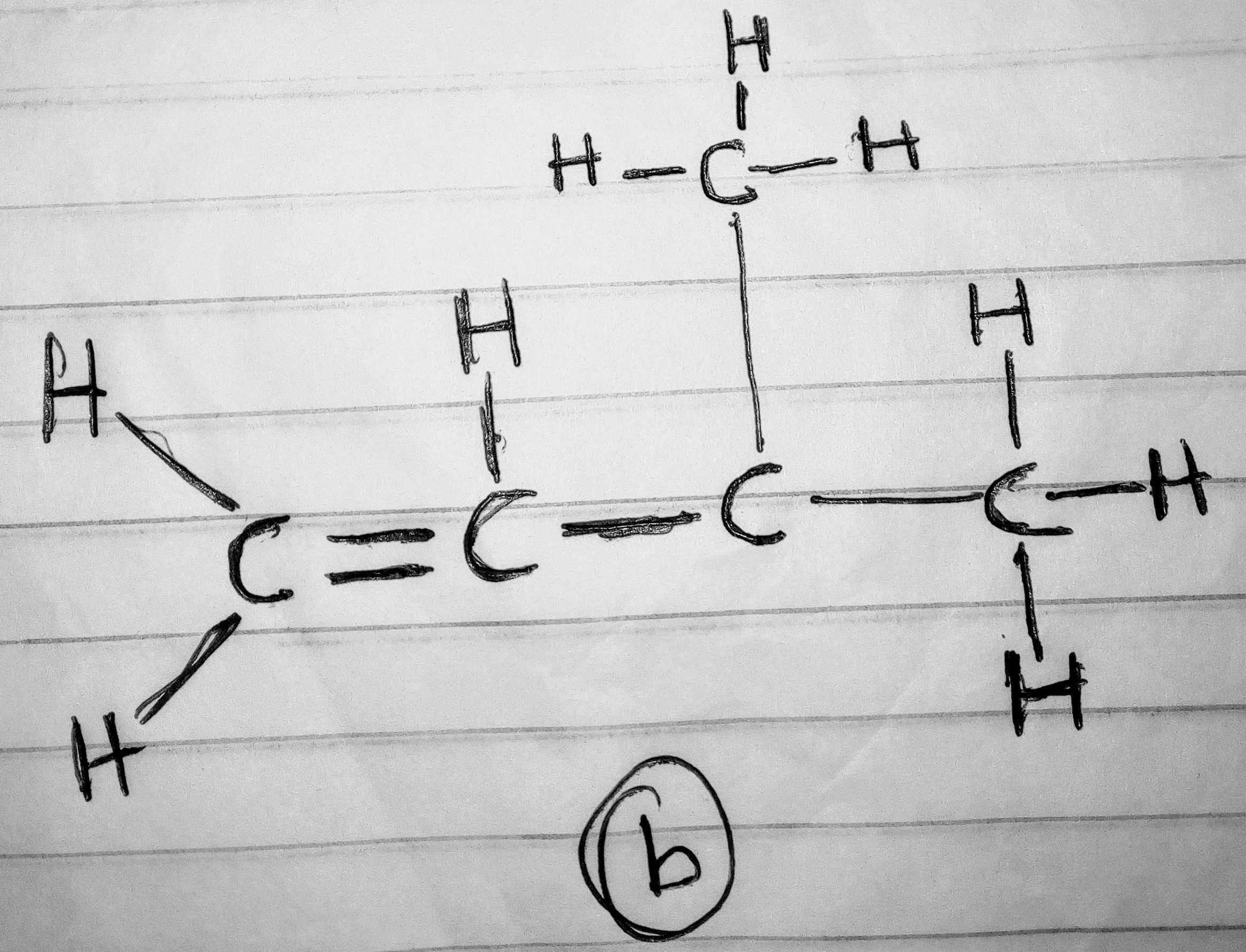Content created and supplied by: studio.opera.com (via Opera News )

Chemistry Revision Hydrogen Nitrogen Organic Organic Chemistry